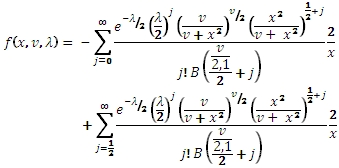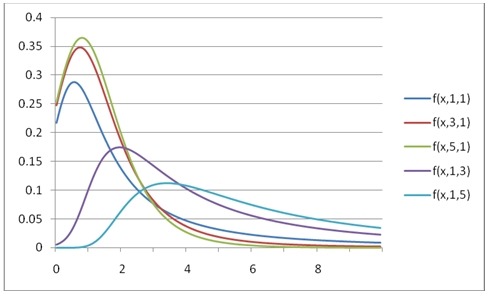# SQL Server non-central T distribution function

NCTPDF

Updated: 31 July 2010

Use NCTPDF to calculate the probability density function of the non-central T distribution.

The formula for the probability density function is:Syntax
SELECT [wctStatistics].[wct].[NCTPDF] (
<@X, float,>
,<@DF, float,>
,<@Lambda, float,>)
Arguments
@X
is the variable to be evaluated. @X is an expression of type float or of a type that implicitly converts to float
@DF
degrees of freedom. @DF is an expression of type float or of a type that implicitly converts to float
@Lambda
is the non-centrality parameter. @Lambda is an expression of type float or of a type that implicitly converts to float
Return Types
float
Remarks
·         @X must be greater than zero (@X > 0).
·         @DF must be greater than zero (@DF > 0).
·         @Lambda must be greater than or equal to zero (@Lambda > 0).
Examples
Calculate the probability density function:
SELECT wct.NCTPDF(1,3,3)
This produces the following result
----------------------
0.0700404713849545

(1 row(s) affected)

You can use the SeriesFloat function from the XLeratorDB/math library to generate a dataset which can be pasted into EXCEL to generate a graph of the porbability density function.
SELECT SeriesValue
,wct.NCTPDF(SeriesValue, 1, 1) as [f(x,1,1)]
,wct.NCTPDF(SeriesValue, 3, 1) as [f(x,3,1)]
,wct.NCTPDF(SeriesValue, 5, 1) as [f(x,5,1)]
,wct.NCTPDF(SeriesValue, 1, 3) as [f(x,1,3)]
,wct.NCTPDF(SeriesValue, 1, 5) as [f(x,1,5)]
FROM wctMath.wct.SeriesFloat(.1, 10, .1,NULL,NULL)
This is an EXCEL-generated graph of the results### SupportCopyright 2008-2020 Westclintech LLC         Privacy Policy        Terms of Service